Next: Cold-Plasma Dispersion Relation Up: Waves in Cold Plasmas Previous: Plane Waves in a

# Cold-Plasma Dielectric Permittivity

In a collisionless plasma, the linearized cold-plasma equations are written [see Eqs. (408)-(411)]: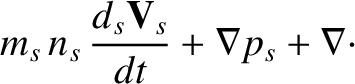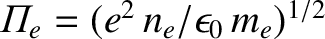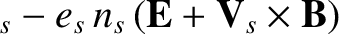(461)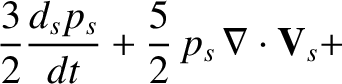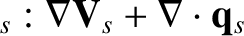(462)

Substitution of plane wave solutions of the type (450) into the above equations yields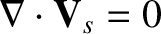(463)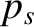(464)

Let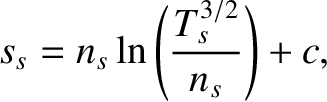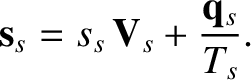(465)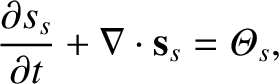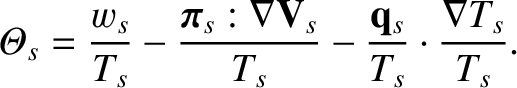(466)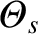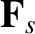(467)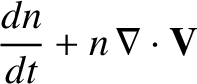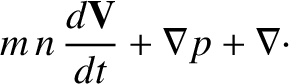(468)

be the electron plasma frequency, the ion plasma frequency, the electron cyclotron frequency, and the ion cyclotron frequency, respectively. The plasma frequency,''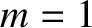, mentioned in Sect. 1, is identical to the electron plasma frequency,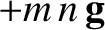. Eliminating the fluid velocitybetween Eqs. (463) and (464), and making use of the above definitions, we obtain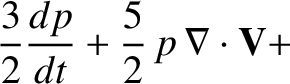(469)

The parallel component of the above equation is readily solved to give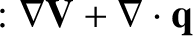(470)

In solving for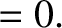, it is helpful to define the vectors: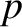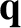(471)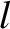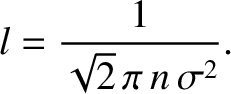(472)

Here,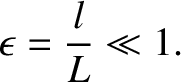are a set of mutually orthogonal, right-handed unit vectors. Note that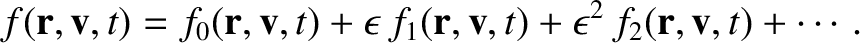(473)

It is easily demonstrated that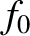(474)

where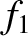, etc.

The conductivity tensor is diagonal in the basis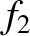. Its elements are given by the coefficients of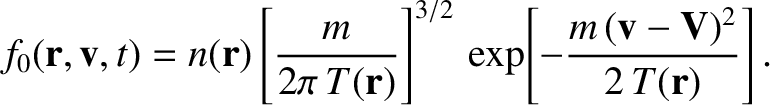and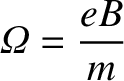in Eqs. (474) and (470), respectively. Thus, the dielectric permittivity (457) takes the form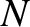(475)

where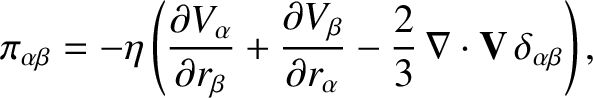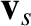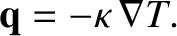(476)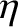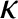(477)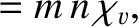(478)

Here,and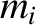represent the permittivities for right- and left-handed circularly polarized waves, respectively. The permittivity parallel to the magnetic field,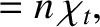, is identical to that of an unmagnetized plasma.

In fact, the above expressions are only approximate, because the small mass-ratio ordering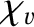has already been folded into the cold-plasma equations. The exact expressions, which are most easily obtained by solving the individual charged particle equations of motion, and then summing to obtain the fluid response, are: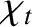(479)(480)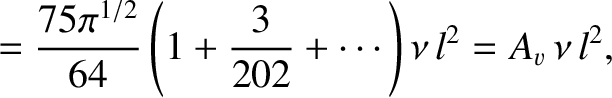(481)

Equations (476)-(478) and (479)-(481) are equivalent in the limit. Note that Eqs. (479)-(481) generalize in a fairly obvious manner in plasmas consisting of more than two particle species.

In order to obtain the actual dielectric permittivity, it is necessary to transform back to the Cartesian basis. Let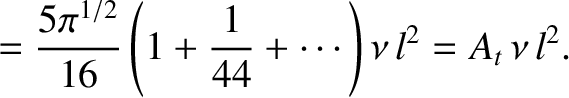, for ease of notation. It follows that the components of an arbitrary vector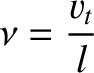in the Cartesian basis are related to the components in the circular'' basis via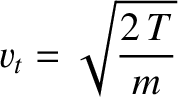(482)

where the unitary matrix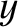is written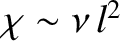(483)

The dielectric permittivity in the Cartesian basis is then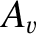(484)

We obtain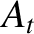(485)

where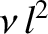(486)

and(487)

represent the sum and difference of the right- and left-handed dielectric permittivities, respectively.Next: Cold-Plasma Dispersion Relation Up: Waves in Cold Plasmas Previous: Plane Waves in a
Richard Fitzpatrick 2011-03-31